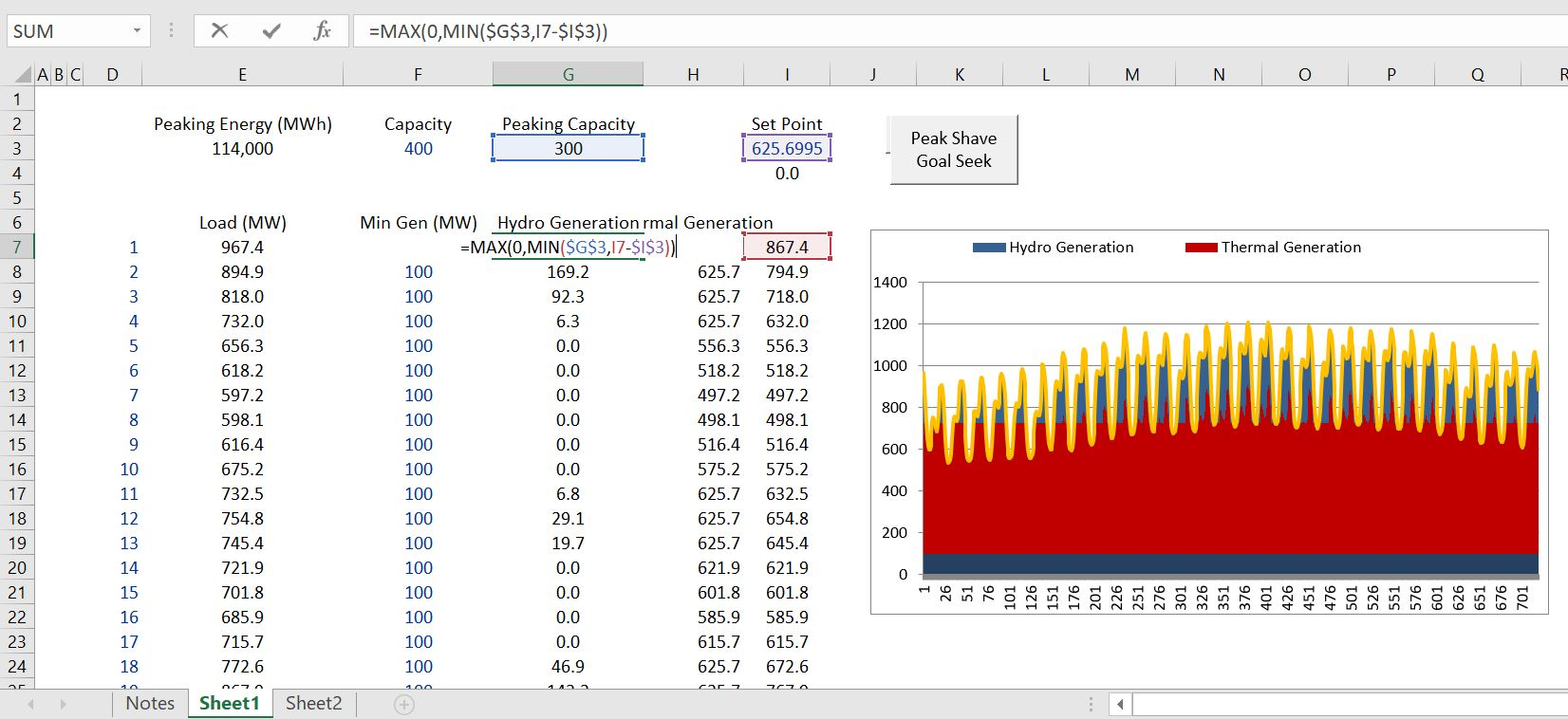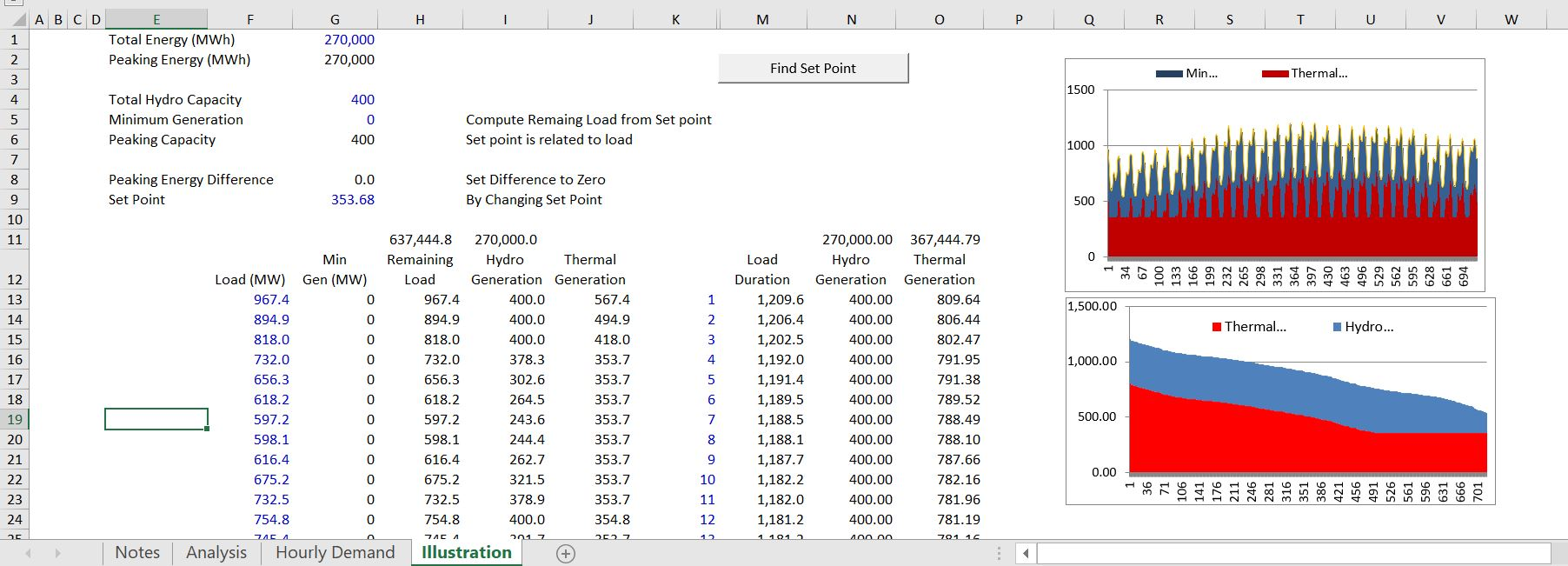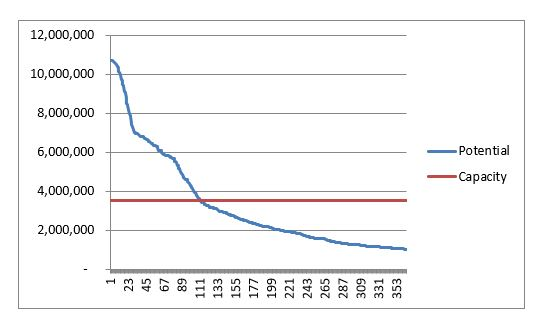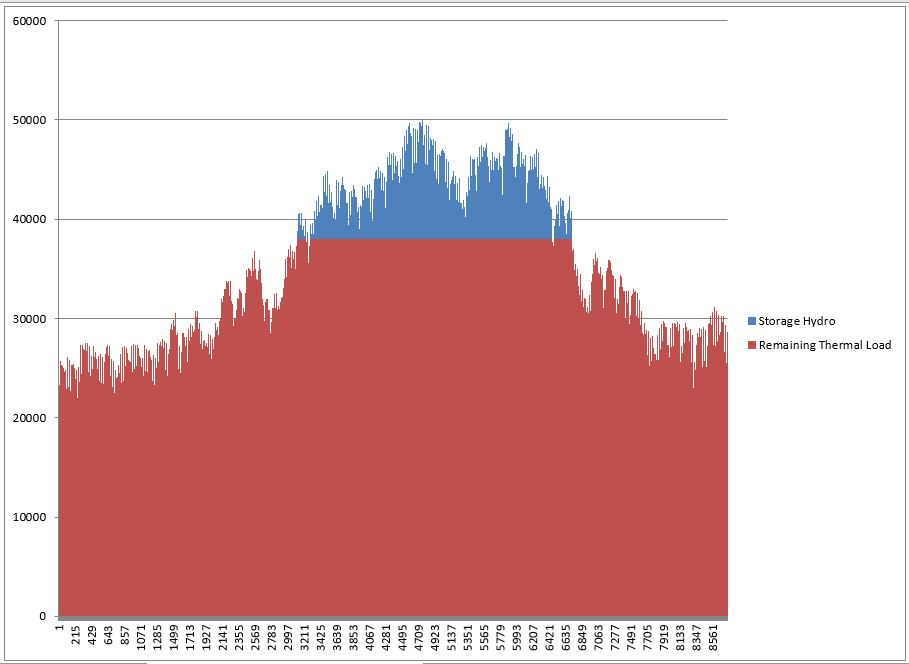# Storage Hydro Peak Shaving

This webpage explains how to model the amount of energy produced by a hydro plant when the plant can store water in a reservoir. The hydro plant has constraints on the amount of energy and the amount of capacity.  Determining the amount of energy to release depends on value of energy at different time periods.  Assuming that the value of energy is directly related to the size of the load, an optimisation process is easy to accomplish using the goal seek tool. To compute peak shaving you can start with the basic load.  Then you put in an arbitrary set point which is the amount of load that is present before which the water is released.  If load is above the set point, energy is produced by the hydro plant. You can sum the amount of energy using the set point.  Then you can make sure that the sum of the energy produced by the hydro plant is equal to the energy available. You should also make sure that the capacity of the generator is not exceeded in any hour.  My good friend Tom Vesalka has taught me this.

The peak shaving algorithm is demonstrated in the file that you can download by pressing the button below. There are just a couple of equations and a goal seek function.  You need a maximum function with the load relative to the hydro generation and to make sure the hydro generation is not negative.  For this you can use a MIN and MAX together as shown on the screenshot below.

The process of using a goal seek function to find the set point is illustrated in the screenshot below. You can compute the difference between the computed generation from the hydro plant and the amount of energy available. This difference should be zero.  To get the difference to zero you should also make an equation with the minimum function which assures that the generation cannot exceed the capacity of the generator.  You need to first define the capacity factor and the capacity of the hydro project.  The production in any hour cannot exceed the capacity and it should be limited by the set point.  In the example below the set-point is in Cell I3.  The capacity factor is defined by the amount of energy which is used in a goal seek.  The goal seek process is illustrated in the second screenshot below.The goal seek macro is simple as illustrated below.  You should name ranges and record a macro.```.

Sub peak_shave()
'
' peak_shave Macro
'
Range("difference").GoalSeek Goal:=0, ChangingCell:=Range("set_point")
End Sub

```

The screenshot below illustrates how you can use the MIN and MAX and a set point to compute the total energy when you are peak shaving.

``The computation of peak shaving is demonstrated in the file that you can download by pressing the button below.